# Escape Velocity Formula

Escape Velocity Formula

The escape velocity is the minimum velocity required to leave a planet or moon. For a rocket or other object to leave a planet, it must overcome the pull of gravity. The formula for escape velocity contains a constant, G, which is called the "universal gravitational constant". Its value is. The unit for escape velocity is meters per second (m/s).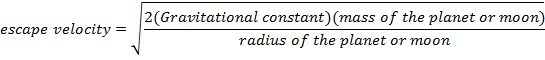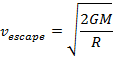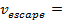escape velocity (m/s)

G = universal gravitational constant ()

M = mass of the planet or moon (kg)

R = radius of the planet or moon (m)

Escape Velocity Formula Questions:

1) The radius of Earth is 6.38x106 m, and the mass of the Earth is 5.98x1024 kg. What is the escape velocity from Earth?

Answer: The escape velocity from Earth can be found using the formula: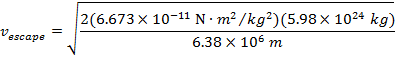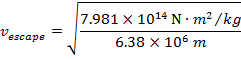11184 m/s

The escape velocity from Earth is 11 184 m/s, or approximately 11.2km/s.

2) To leave the moon, the Apollo astronauts had to take off in the lunar module, and reach the escape velocity of the moon. The radius of the moon is 1.74x106 m, and the mass of the moon is 7.35x1022 kg. What velocity did Neil Armstrong and Buzz Aldrin in the lunar module have to reach to leave the moon?

Answer: The escape velocity from the moon can be found using the formula: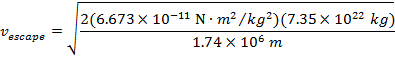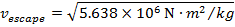2374 m/s

The escape velocity from the moon is 2374 m/s, or approximately 2.37 km/s.

 Related Links: Velocity Practice Problems Quiz Speed and Velocity Quiz Momentum Examples Speed vs. Velocity Velocity Formula Angular Velocity Formula Orbital Velocity Formula Initial Velocity Formula